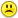+0

# Here are 2 number puzzles for you.

+5
1186
17

Here is 2 number puzzle for you.

*The first one is tough, the second one is easier.

You do not have to use every number but you cannot use any number twice.

You can only use + - x and divide and brackets.

If you answer make sure you repeat the question so we all know what you are answering!---------------------------------------------------------

(1)       2,8,25,50,75,100  Magic number is 431

---------------------------------------------------------

Here is an easier one.

There are at least 3 different solutions to this one!

(2)       4,6,25,50,75,100   Magic number is 821

----------------------------------------------------------

Aug 5, 2014

#16
+5

and for the hard one I got

(50*75-(100+2))/8-25=431

Sep 9, 2014

#1
0

solution:

OR Melody's way    6(100+50)-75-4 = 821

Aug 5, 2014
#2
+3

(1)       2,8,25,50,75,100  Magic number is 431

Couldn't solve...

Aug 5, 2014
#3
+3

(2)       4,6,25,50,75,100   Magic number is 821

Couldn't solve...

Aug 5, 2014
#4
+3

Hi Ninja,

Somehow I think your first solution needs some brackets?  No it still won't work.  Plus you need to swap the 4 for 8/2Why don't you try the easier one?  The hard one is REALLY difficult.

Aug 5, 2014
#5
+3

Yeah I messed up a little, I think I copied the wrong set of numbers for the wrong problem or something.

I guess these were harder than they looked!

I just edited my posts a little. Sorry to messy up your thread.Aug 5, 2014
#6
0

---------------------------------------------------------

(1)       2,8,25,50,75,100  Magic number is 431

---------------------------------------------------------

$$\left[\frac{75 + 25}{50}+2 \right]*\left[100+8 \right]\Rightarrow$$ For this one, 431/108 is 3.99 but can it be rounded to 4?

$$\left[\frac{100+8}{\frac{75}{2}-25}\right]*50\rightarrow$$ For this one, rats...result is 8.64 after dividing the first part; too bad it can't be 8.62! Does it have to be the exact number or can it be rounded to 8.6...if so, then 431.5!

Aug 9, 2014
#7
+3

In the kingdom of Camelot we strive for perfection.  I am using a small magical pigeon so I have not given your answer the  consideration it deserves. However, an exact answer be possible in this magical Kingdom :)

Queen of Camelot.

Aug 9, 2014
#8
0

(1)       2,8,25,50,75,100  Magic number is 431

$$\boxed{\dfrac{\left(50-{\dfrac{100}{25}}\right)*75-2}{8} = 431}$$

$$\boxed{\dfrac{50*75-2-100}{8}-25 = 431}$$

$$\boxed{\left( 100 + \dfrac{75}{50} \right)*\left( \dfrac{8}{2}\right) = 431}$$

.
Aug 21, 2014
#9
0

(2)       4,6,25,50,75,100   Magic number is 821

$$\dfrac{(6+4*50)*100-75}{25} = 821$$

$$\left( 50+6 \right) \left( \dfrac{4*100}{25}\right)-75 = 821$$

$$\left( 50-6 \right) \left( \dfrac{25*75}{100}\right)-4 = 821$$

$$\left( \dfrac{25*75}{50}+100\right)*6-4 = 821$$

$$\dfrac{( 75-25)*100-50 }{6}-4 = 821$$

$$\dfrac{( 50*100+25-75}{6}-4 = 821$$

$$50-4+25*(6-75+100) = 821$$

$$75-4+6*(100+50-25) = 821$$

$$100-4-25+6*(50+75) = 821$$

.
Aug 21, 2014
#10
0

I think I got a different one again for the 821 magic number one.

Anyway here are the solutions that I had.  They have been hiding all this time on an invisable post :)

These videos were  from an Australian show called "Letters and Numbers"

Lily Serna is awesome.another solution to 821 is  also really 'out there'http://web2.0calc.com/questions/here-is-a-number-puzzle-for-you-nbsp-it-s-a-tough-one#r1

Aug 21, 2014
#11
0

Hi Melody,

6(100+50) - 75 - 4 = 821

But what about Number 25  (4,6,25,50,75,100) ?

(100+25)*6 + 75 - 4 = 821

But what about Number 50  (4,6,25,50,75,100)  ?Aug 21, 2014
#12
0

Hi Heureka,

Here are the rules listed at the top of the post

You do not have to use every number but you cannot use any number twice.

So you found all those wonderful solutions and you were making the question harder than it even needed to be.Aug 21, 2014
#13
+5

Hi Melody,

thank you for the rules.

(1)       2,8,25,50,75,100  Magic number is 431

five Numbers:

$$\left( \dfrac{75}{100}+50 \right)*8+25=431$$

(2)       4,6,25,50,75,100   Magic number is 821

five Numbers:

6*(100+50) - 75 - 4 = 821  ( your solution )

6*(100+25) + 75 - 4 = 821 ( your solution )Aug 22, 2014
#14
0

You are getting me a little confused Heureka.  Don't worry about it though.  I spend my whole life in a state of confusion. lol.

Aug 22, 2014
#15
0

here is what I came up with for the easy one :)

6(100+50) - 75 - 4 = 821

Sep 9, 2014
#16
+5

and for the hard one I got

(50*75-(100+2))/8-25=431